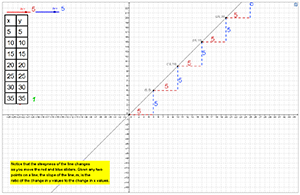Slope

My Definition

Key Characteristics

The following are true of slope:

• It describes the steepness of a line.

• It can also be referred to as the rate of change.

• It can be written as a ratio that compares the change in the dependent variable to change in the independent variable,
ΔyΔx
.

• It can be positive, negative, zero, or undefined.

• It is represented algebraically by the variable, m, in the formula y = mx + b.

• It can be determined using the formula m =
y2 - y1x2 - x1
when two points on a line are known.

• It can be determined using the sides of right triangles (slope triangles) on a graph.

Example

Use this link below to further study an example of slope.

Slope ActivityNon-example

Given the graph of y =
23
x + 4, the y-intercept is 4.TEKS: 7(7)(A), 8(4)(A), 8(4)(B), 8(4)(C)